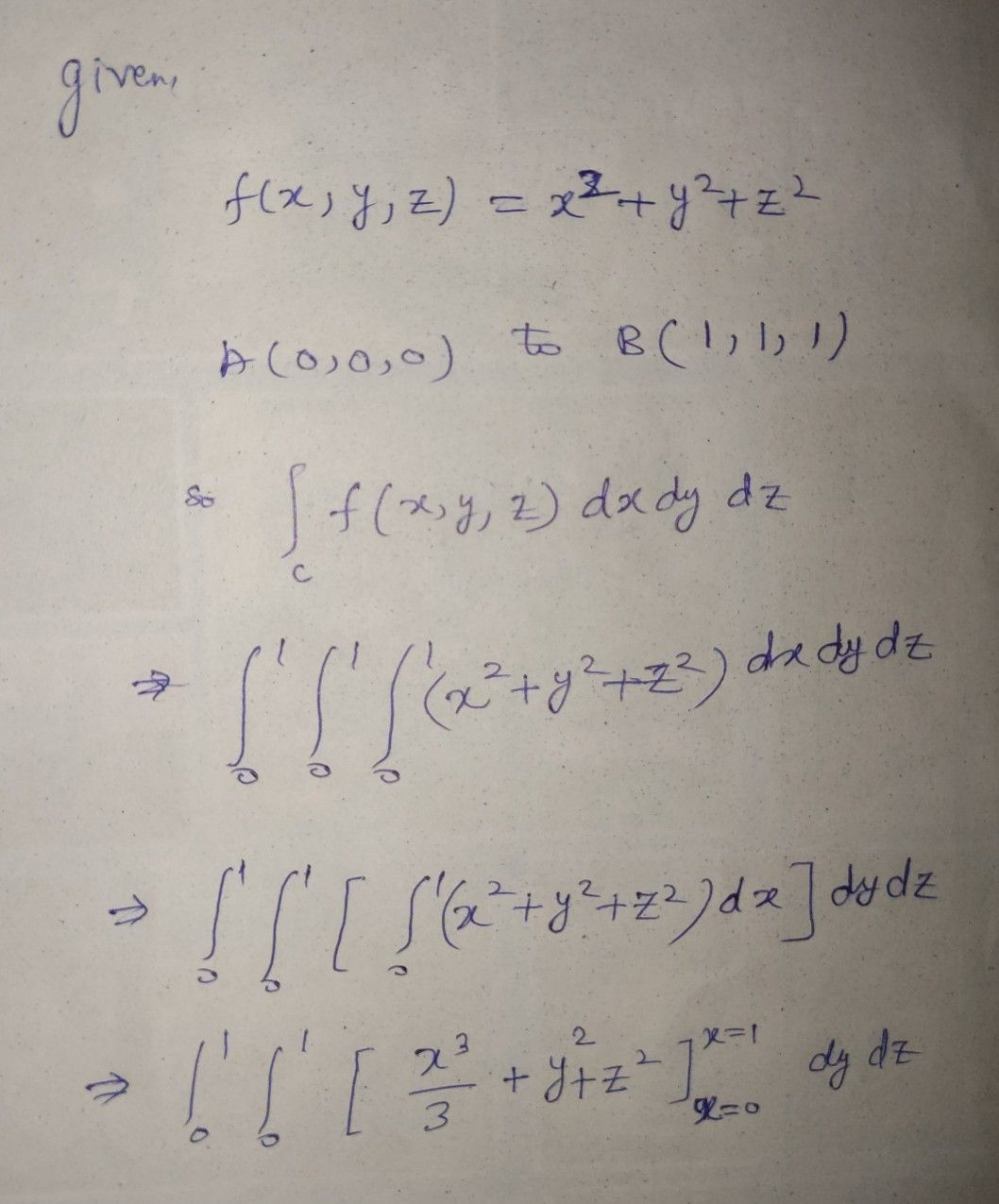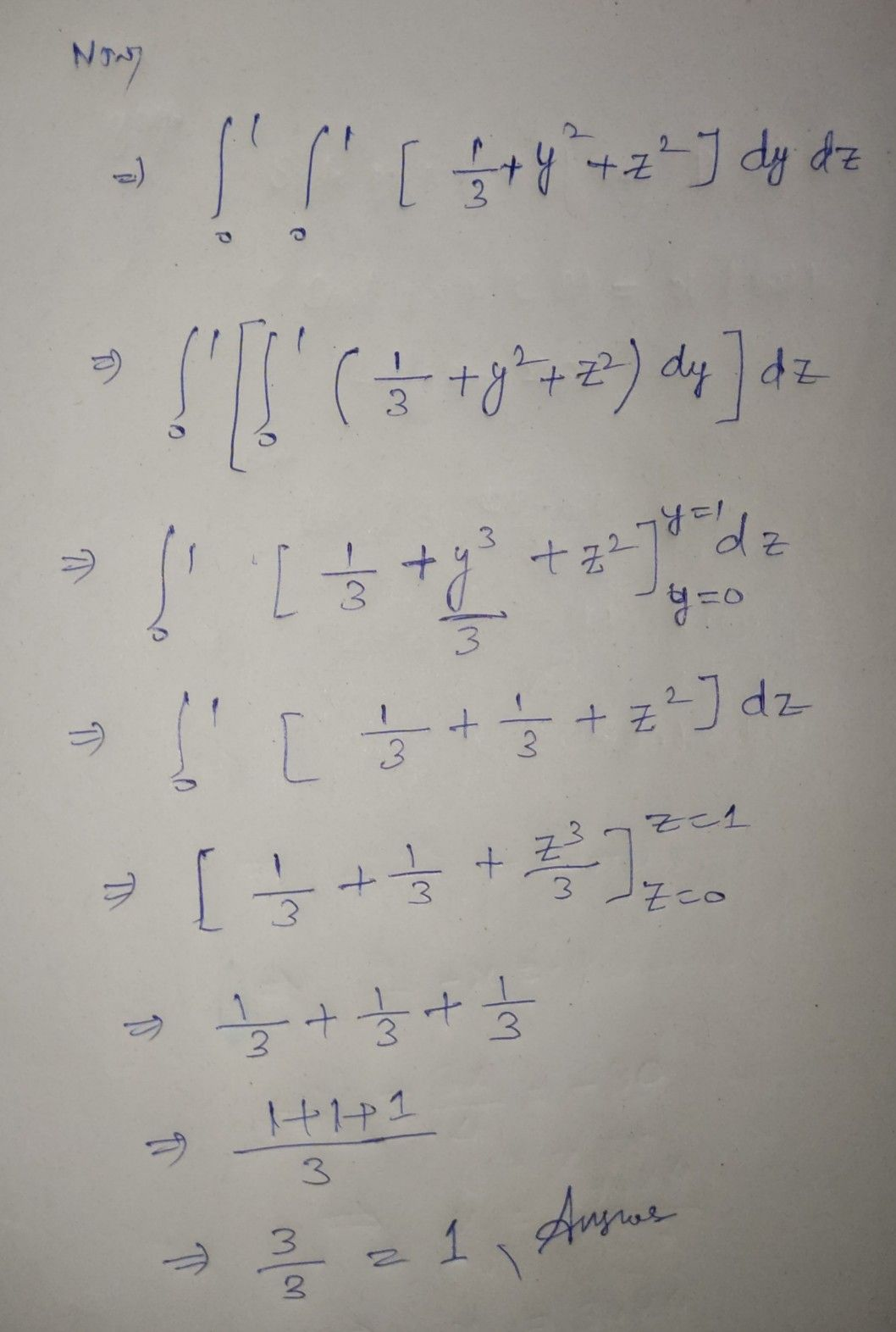Symbol
ProblemLet $C$ be the straight line joining the points $A\left(0.0.0\right)$ $10B\left(1,1.1\right)$ Then the line integration of $f\left(x.y.z\right)=x^{2}+y^{2}+z^{2}$ over the $c$ is
Calculus
Search count: 109
SolutionQanda teacher - Mashroorplease evaluate, and give some extra Coins as thank you gift
if any doubts kindly ask will clarify